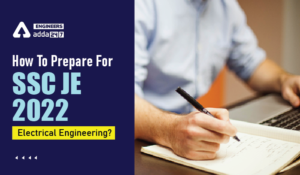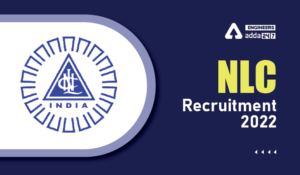Engineering Jobs   »   Mechincal Engineering quizs   »   NHPC JE Recruitment 2021, NHPC JE...

# NHPC-JE’21 ME: Daily Practices Quiz 21-Sep-2021

## NHPC JE Recruitment 2021

National Hydroelectric Power Corporation (NHPC) has released the recruitment notification for engagement of following posts such as Senior Medical Officer, Assistant Rajbhasha Officer, Junior Engineer & Sr. Accountant posts. NHPC expects application from High Performing, Dynamic & Achievement-Oriented Professionals candidates to fill up these 173 vacancies.

## NHPC-JE’21 ME: Daily Practices Quiz

NHPC-JE’21 ME: Daily Practices Quiz 21-Sep-2021

Quiz: Mechanical Engineering

Exam: NHPC JE
Topic: SOM

Each question carries 1 mark
Negative marking: 1/4 mark
Time: 8 Minutes

Q1. Maximum shear stress theory was postulated by ___.
(a) St. Venant
(b) Mohr
(c) Rankine
(d) Tresca

Q2. Which of the following is TRUE for factor of safety?
(a) Tensile stress/permissible stress
(b) Compressive stress/Ultimate stress
(c) Ultimate stress/Permissible stress
(d) Ultimate stress/Shear stress

Q3. A body is subjected to principle stresses at a point having value as 200 MPa, 150 MPa and 100 MPa respectively. what is the value of maximum shear stress in (MPa)
(a) 25
(b) 50
(c) 75
(d) 100

Q4. A solid shaft transmits a torque T. the allowable shear stress is τ. The diameter of
(a) ∛(16T/πτ)
(b) ∛(32T/πτ)
(c) ∛(4T/πτ)
(d) ∛(64T/πτ)

Q5. The intensity of stress which causes unit strain is called ___________.
(a) unit stress
(b) bulk modulus
(c) modulus of rigidity
(d) modulus of elasticity

Q6. The rate of change of bending moment is equal to
(a) shear force at that section
(b) deflection at the section
(d) slope at that section

### Solutions

S1. Ans.(d)
Sol. maximum shear stress theory also known as Guest & Tresca theory because it is postulated by Tresca.

S2. Ans.(c)
Sol. factor of safety is defined as the ratio of failure stress (i.e. ultimate stress) to the working stress (i.e. permissible stress)
FOS=(Ultimate stress)/(Permissible stress)

S3. Ans.(b)
Sol. Given
σ_1=200 MPa,σ_2=150 MPa,σ_3=100 MPa
Maximum shear stress
((σ_1- σ_2)/2) or =((σ_2- σ_3)/2) or ((σ_3- σ_1)/2)
So, ((200-100)/2)=50 MPa
S4. Ans.(a)
Sol. we know that, the torsion equation
τ/J=τ/r=Gθ/L
T/J=τ/r [J=π/32 d^4 ]
T/(π/32 d^4 )=τ/(d\/2)
τ=16T/(πd^3 )
d^3=16T/πτ
d=∛(16T/πτ)
S5. Ans.(d)
Sol. The intensity of stress which causes unit strain is called modules of elasticity.

S6. Ans.(a)
Sol. The rate of change of bending moment is equal to shear force at that section.
Let M is the bending moment at x–x section and f is the shear force at x-x section
▭((dm)_(x-x)/dx=f_(x-x) )

Sharing is caring!

•SSC JE 2022 Apply Online, Link Activated...
•How to Clear SSC JE 2022 Electrical Engi...
•JKPSC AE Cutoff 2022, Check Here to Know...
•NLC Recruitment 2022, Apply Online for 9...
•SSC JE Post Preference, Check the SSC JE...
•SSC JE 2022 Notification, Check here the...